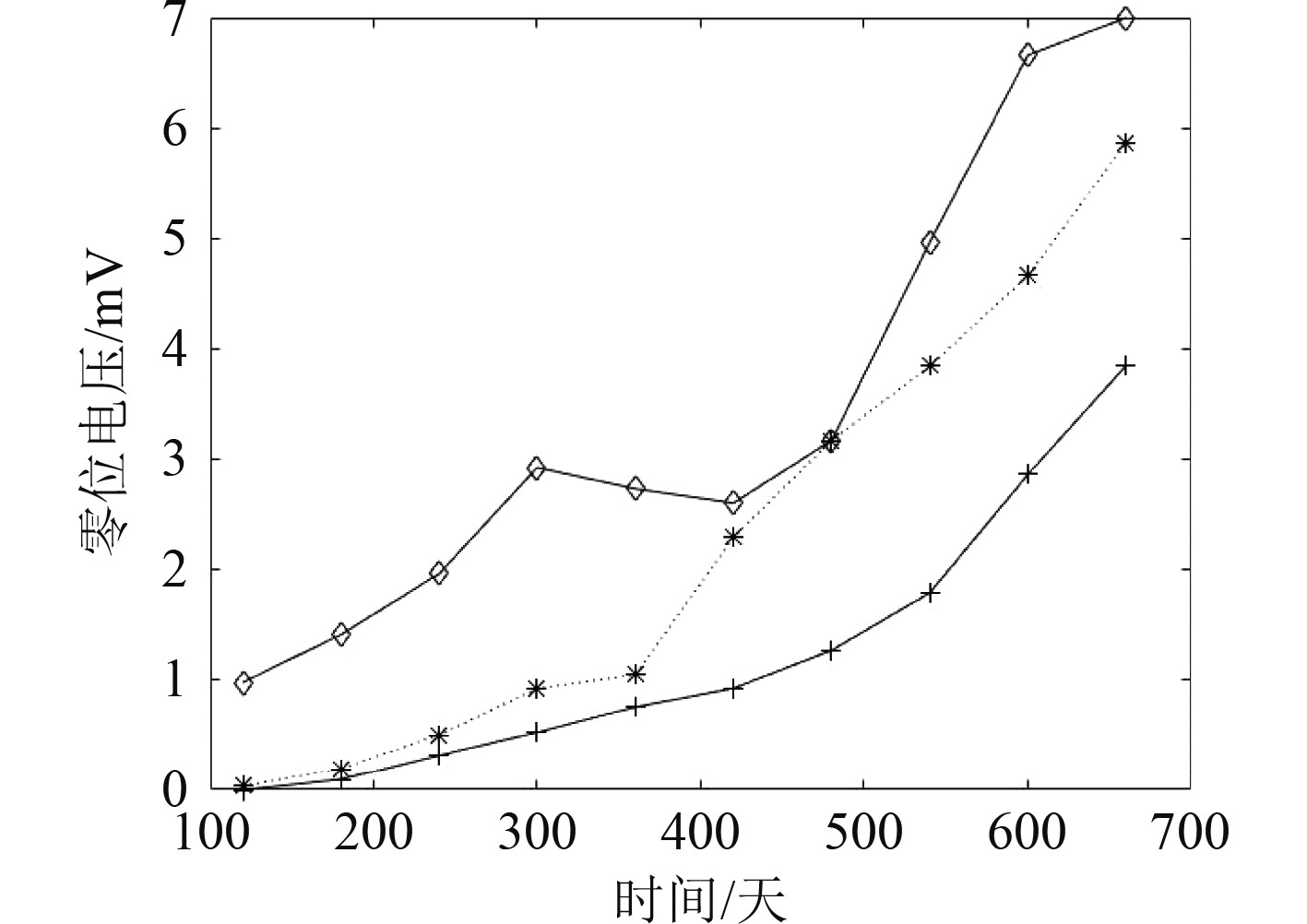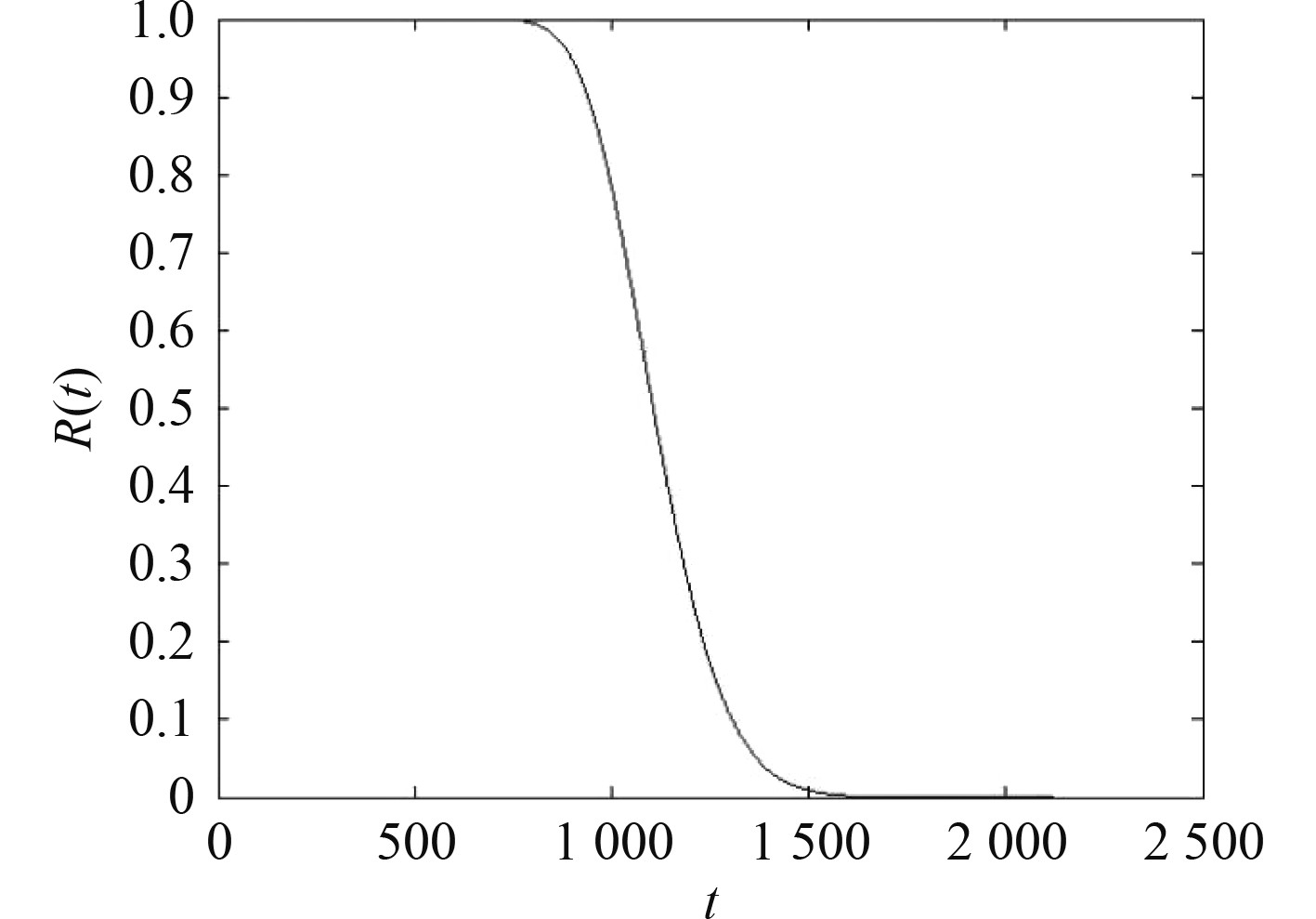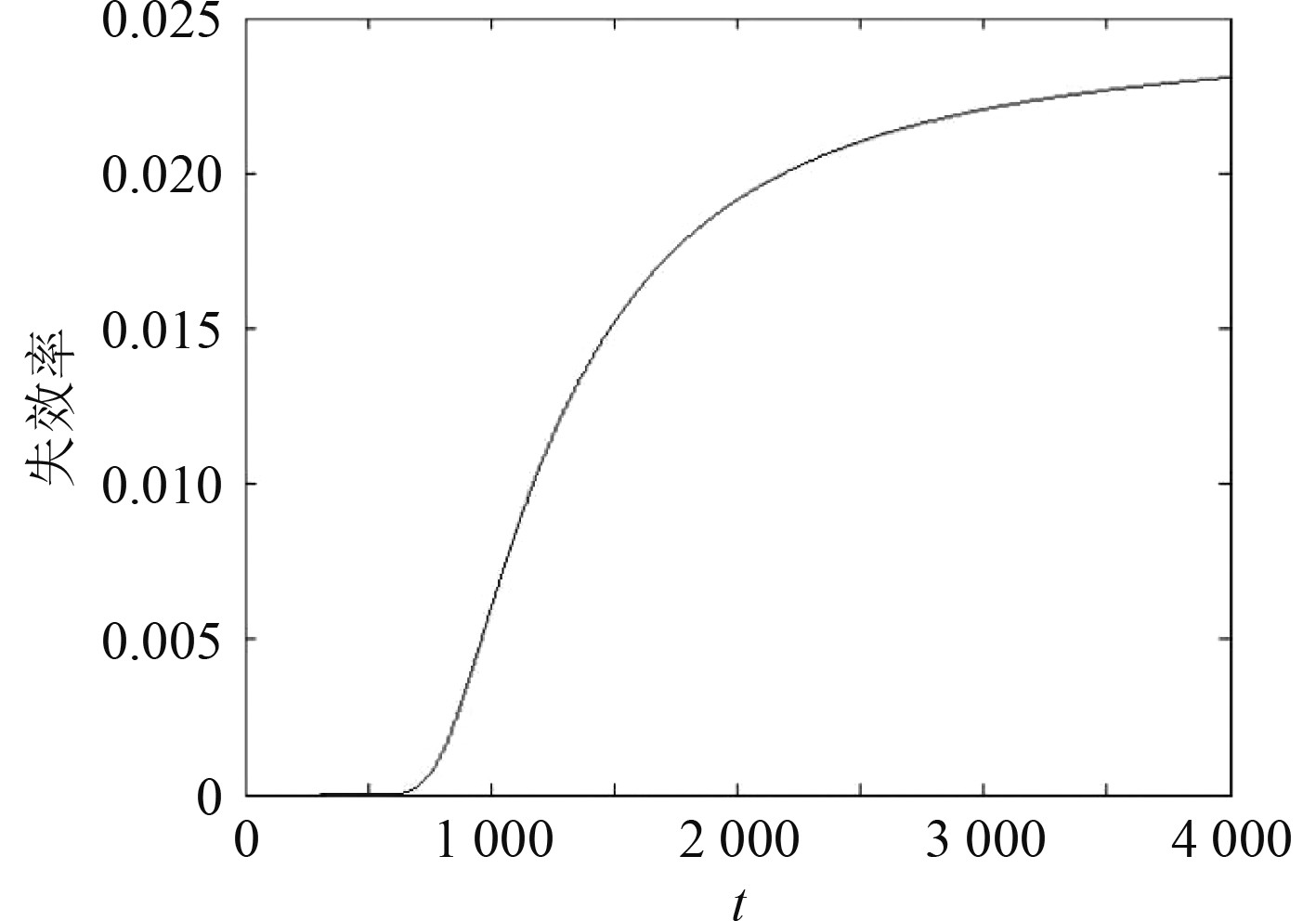﻿ 基于冲击的舰炮设备可靠性建模与评估
 舰船科学技术2022, Vol. 44Issue (14): 165-169    DOI: 10.3404/j.issn.1672-7649.2022.13.035PDF

Research on reliability modeling and assessment of naval gun equipment based on impact theory
The 713 Research Institute of CSSC, Zhengzhou 450015, China
Abstract: Under certain assumptions, the reliability model of naval gun equipment based on impact factors is established. The features of reliability functions and failure rates are analyzed and the reliability evaluation method is put forward. In the reliability evaluation method, the parameters in the equipment reliability model are estimated by using the performance degradation information and the fault information. Finally, an example is given to illustrate the applicability of the model and the effectiveness of the evaluation method in characterizing the reliability of naval gun equipment.
Key words: naval gun equipment     reliability modeling     reliability assessment     degradation data     impact theory
0 引　言

1 舰炮设备可靠性影响因素分析

2 舰炮设备可靠性模型 2.1 模型假设

 $P\left( {{N_1}(t) = n} \right) = \frac{{{{\left( {\lambda t} \right)}^n}}}{{n!}}\exp \left( { - \lambda t} \right)\text{，}n = 1,2, \cdots，$ (1)

 $P\left( {{N_2}(t) = n} \right) = \frac{{{{\left( {at} \right)}^n}}}{{n!}}\exp \left( { - at} \right)\text{，}n = 1,2, \cdots 。$ (2)

2.2 舰炮设备寿命及其分布

1）考虑致命冲击流导致的设备硬故障。

 $\begin{split} {F_1}(t) = P\left( {{T_1} \leqslant t} \right) & = 1 - P\left( {{T_1} > t} \right) = 1 - P\left( {{N_1}(t) = 0} \right) =\\ & 1 - \exp ( - \lambda t) 。\\[-10pt] \end{split}$ (3)

2）考虑非致命冲击流对设备性能指标的影响

 $X(t) = \sum\limits_{i = 1}^{{N_2}(t)} {{Y_i}}。$ (4)

 $X(t) \sim N\left( {{\mu _t},\sigma _t^2} \right)。$ (5)

 ${T_2} = \min \left( {t:\inf \left\{ {t:X(t) = {X_{cu}},t \geqslant 0} \right\}} \right) ，$

 $\begin{split} {F_2}\left( t \right) = P\left\{ {{T_2} \leqslant t} \right\} & = 1 - P\left\{ {{T_2} > t} \right\} = 1 - P\left\{ {\;X(t) \leqslant {X_{cu}}} \right\} = \\ & 1 - \Phi \left( {\frac{{{X_{cu}} - {\mu _t}}}{{{\sigma _t}}}} \right) 。\\[-20pt] \end{split}$ (6)

 $\begin{split} F\left( t \right) = P\left\{ {T \leqslant t} \right\} &= P\left\{ {\min ({T_1},{T_2}) \leqslant t} \right\} = 1 - P\left\{ {{T_1} > t,{T_2} > t} \right\} =\\ & 1 - \left[ {1 - {F_1}(t)} \right]\left[ {1 - {F_2}(t)} \right] =\\ & 1 - \exp \left( { - \lambda t} \right)\varPhi \left( {\frac{{{X_{cu}} - {\mu _t}}}{{{\sigma _t}}}} \right) 。\\[-20pt] \end{split}$ (7)
2.3 舰炮设备可靠性模型

 $\begin{split} R\left( t \right) = P\left\{ {T > t} \right\} &= P\left\{ {\min ({T_1},{T_1}) > t} \right\}= \\ & \left[ {1 - {F_1}(t)} \right]\left[ {1 - {F_2}(t)} \right] =\\ & \varPhi \left( {\frac{{{X_{cu}} - {\mu _t}}}{{{\sigma _t}}}} \right)\exp \left( { - \lambda t} \right) 。\\[-10pt] \end{split}$ (8)

 $\begin{split} {\lambda _s}\left( t \right)& = - \frac{{R'(t)}}{{R(t)}} = \lambda - \frac{{{\rm{d}}\varPhi \left( {({X_{cu}} - {\mu _t})/{\sigma _t}} \right)}}{{{\rm{dt}}}}{\varPhi ^{ - 1}}\left( {\frac{{{X_{cu}} - {\mu _t}}}{{{\sigma _t}}}} \right) =\\ & \lambda + \left( {\frac{{{X_{cu}}}}{{2t}} + \frac{{a\mu }}{2}} \right){e^{ - \frac{{{{\left( {{X_{cu}} - a\mu t} \right)}^2}}}{{2a({\mu ^2} + {\sigma ^2})t}}}}{\left[ {\int_{ - \infty }^{{X_{cu}}} {{e^{ - \frac{{{{\left( {{X_{cu}} - a\mu t} \right)}^2}}}{{2a({\mu ^2} + {\sigma ^2})t}}}}} {\rm{d}}x} \right]^{ - 1}} 。\\[-20pt] \end{split}$ (9)

 $\begin{split} & \mathop {\lim }\limits_{t \to + \infty } {\lambda _s}\left( t \right)=\\ &\;\; \lambda + \mathop {\lim }\limits_{t \to + \infty } \left( {\frac{{{X_{cu}}}}{{2t}} + \frac{{a\mu }}{2}} \right){e^{ - \frac{{{{\left( {{X_{cu}} - a\mu t} \right)}^2}}}{{2a({\mu ^2} + {\sigma ^2})t}}}}{\left[ {\int_{ - \infty }^{{X_{cu}}} {{e^{ - \frac{{{{\left( {{X_{cu}} - a\mu t} \right)}^2}}}{{2a({\mu ^2} + {\sigma ^2})t}}}}} {\rm{d}}x} \right]^{ - 1}} =\\ &\;\; \lambda + \frac{{a\mu }}{{2\sqrt {a({\mu ^2} + {\sigma ^2})} }}{e^{\frac{{a\mu {X_{cu}}}}{{({\mu ^2} + {\sigma ^2})}}}}\mathop {\lim }\limits_{t \to + \infty } \frac{{{e^{ - \frac{{a{X_{cu}}}}{{({\mu ^2} + {\sigma ^2})}}}}}}{{\sqrt t \int_{ - \infty }^{\frac{{{X_{cu}} - a\mu t}}{{\sqrt {a({\mu ^2} + {\sigma ^2})t} }}} {{e^{ - \frac{{{x^2}}}{2}}}} {\rm{d}}x}} ，\end{split}$

 ${\lambda _s} = \mathop {\lim }\limits_{t \to + \infty } {\lambda _s}\left( t \right) = \lambda + \frac{a}{2}\left( {\frac{{{\mu ^2}}}{{{\mu ^2} + {\sigma ^2}}}} \right)。$ (10)

3 舰炮设备可靠性评估

3.1 可靠性信息结构

 $\left( {{t_i},{X_{ij}}} \right),i = 1,2, \cdots ,m,j = 1,2, \cdots ,n 。$ (11)

3.2 模型参数估计

 $\hat \lambda = \left\{ \begin{gathered} \frac{T}{r},\quad \quad r > 0 ，\\ T,\quad \quad r = 0 。\\ \end{gathered} \right.$ (12)

 $X\left( t \right) \sim N\left( {ut,vt} \right)，$ (13)

 ${L_j}\left( {b,u,v} \right) = \prod\limits_{i = 1}^m {\frac{1}{{\sqrt {2\text{π} vt_j^{}} }}\exp \left( { - \frac{{{{\left( {{X_{ij}} - ut_j^{}} \right)}^2}}}{{2vt_j^{}}}} \right)}。$

 $\left\{ \begin{gathered} \hat u = {{\sum\limits_{j = 1}^n {\sum\limits_{i = 1}^m {{X_{ij}}} } } \mathord{\left/ {\vphantom {{\sum\limits_{j = 1}^n {\sum\limits_{i = 1}^m {{X_{ij}}} } } {\sum\limits_{j = 1}^n {mt_j^{}} }}} \right. } {\sum\limits_{j = 1}^n {mt_j^{}} }}，\\ \hat v = \frac{1}{{mn}}\sum\limits_{j = 1}^n {\sum\limits_{i = 1}^m {\frac{{{{\left( {{X_{ij}} - t_j^{}u} \right)}^2}}}{{t_j^{}}}} } 。\\ \end{gathered} \right.$ (14)
3.3 可靠性指标估计

 ${\hat R^*}(t) = \varPhi \left( {\frac{{{X_{cu}} - \hat ut}}{{\sqrt {\hat vt} }}} \right)\exp \left( { - \hat \lambda t} \right)，$ (15)

 ${\hat \lambda _s} = \hat \lambda + \frac{{{{\hat u}^2}}}{{2\hat v}}。$ (16)
4 实例分析

1）致命冲击参数 $\lambda$ 的估计。

2）退化数据的预处理图 1 传感器零位电压随时间变化趋势 Fig. 1 The zero voltage of the sensor changes with time

3）退化模型参数估计

4）可靠度与故障率的估计图 2 传感器的可靠度曲线图 Fig. 2 Reliability curve of the sensor图 3 传感器的故障率曲线图 Fig. 3 Failure rate curve of the sensor
 $\begin{split} {\hat \lambda _s} =& \hat \lambda + \frac{{{{\hat u}^2}}}{{2\hat v}} = 4.6512 \times {10^{ - 4}}+ \\ &{(3.7917 \times {10^{ - 4}})^2}/(2 \times 7.0833 \times {10^{ - 5}})=\\ & 1.4798 \times {10^{ - 3}} 。\end{split}$

5 结　语

  翟亚利. 维修条件下的舰船装备性能退化可靠性建模及其应用研究[D]. 武汉: 海军工程大学, 2019  JI H C, LEE E Y. An extended stochastic failure model for a system subject to random shocks[J]. Operations Research Letters. 2010, 38(5): 468−473.  J. A. M VAN DER W, PANDEY M D. Stochastic analysis of shock process and modeling of condition-based maintenance[J]. Reliability Engineering & System Safety. 2011(96): 619−626.  LU J C, PARK J, YANG Q. Statistical inference of a time-to-failure distribution derived from linear degradation data[J]. Technometrics, 1997, 39: 391-400. DOI:10.1080/00401706.1997.10485158  MARLOW D R, BEALE D J, MASHFORD J S. Risk-based prioritization and its application to inspection of valves in the water sector[J]. Reliability Engineering & System Safety. 2012(100): 67−74.  KALLEN M J. Modelling imperfect mainten- ance and the reliability of complex systems using superposed renewal processes[J]. Reliability Engineering & System Safety. 2011(96): 636−641.  XU Z G, JI Y D, ZHOU D H. Real-time reliability prediction for a dynamic system based on the hidden degradation process identification[J]. IEEE Transaction on Reliability, 2008, 57(2): 230-242. DOI:10.1109/TR.2008.916882  WANG X. Wiener processes with random effects for degradation data[J]. Journal of Multivariate Analysis, 2010, 101: 340-351. DOI:10.1016/j.jmva.2008.12.007  钟强晖, 张志华, 梁胜杰. 基于多元退化数据的可靠性分析方法[J]. 系统工程理论与实践, 2011, 31(3): 544-551. ZHONG Q H, ZHANG Z H, LIANG S J. Reliability analysis approach based on multivariate degradation data[J]. Systems Engineering-Theory & Practice, 2011, 31(3): 544-551. DOI:10.12011/1000-6788(2011)3-544  HUANG T T, WANG L, JIANG T M. Prognostics of products using time series analysis based on degradation data[C]// Prognostics & System Health Management Conference, 2010.  HAO S, YANG J. Reliability analysis for dependent competing failure processes with changing degradation rate and hard failure threshold levels[J]. Computers & Industrial Engineering, 2018, 63(4): 1227-1234.  翟亚利, 张志强, 钟强晖. 具有参数漂移特征的退化可靠性评估方法[J]. 海军工程大学学报, 2013, 25(2): 107−112. ZHAI Y L, ZHANG Z Q, ZHONG Q H. Degradation reliability evaluation method of products with parameters digression feature. Journal of Naval University of Engineering, 2013, 25(2): 107−112.  WANG X L, JIANG P, GUO B, et al. Real-time reliability evaluation based on damaged measurement degradation data[J]. Journal of Central South University. 2012, 19(11): 3162−3169.  CHEN C. Using geometric Poisson exponentially in a compound Poisson production environment weighted moving average control schemes in a compound Poisson production environment[J]. Computers and Industrial Engineering. 2012, 63(2): 374−381.